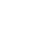# By any means tenable or Untenable

Thermodynamics is the most important branch of the science dealing with the energy, work and conversions of one form of energy into other forms.

It cuts across many branches of the courses like physics, chemistry and engineering. All the students in these streams have to come face to face with this subject.

Its laws have become so perfect that they can be bound into mathematical equations. First law of thermodynamics is concerning the conservation of energy.

Suppose there are two systems which are having energies A & B. If they are connected, then the energy of the resultant system shall be A+B.

But there is a catch. This law does not impose any restrictions on the donors and recipients of the energy. For example, we can heat the water by extracting (suppose we are having such an extracting machine) heat from the ice.

After all the total has to remain constant and sum of the energies of two systems. But the common experience is contrary to this. Heat always flow from higher temperature to lower temperature.

To address this difficulty, second law of thermodynamics was proposed. This takes into account the natural phenomena. Another thermodynamic function called “Entropy” was formulated. This is the measure of randomness of the system. All the systems in nature tends towards chaos or randomness.